Author: Oscar Cronquist Article last updated on February 13, 2023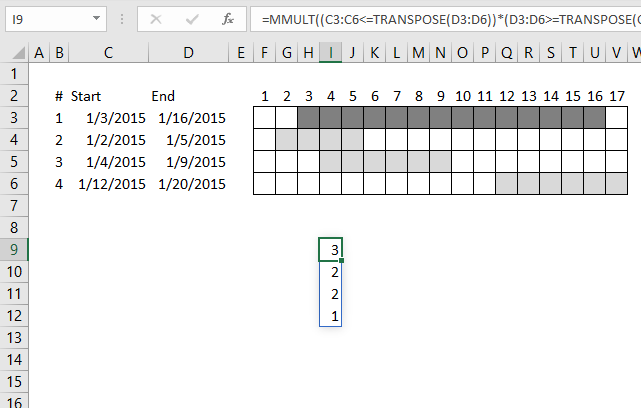This article demonstrates formulas that calculate the number of overlapping ranges for all ranges, finds the most overlapped range and the least overlapped range.

Today's blog post is about date ranges, the techniques demonstrated here can also be applied to Excel time values or other numerical ranges.

The MMULT function is a great excel function, it allows you to do really amazing calculations with date ranges. Yes, I have said that before.

## 1. Calculate the number of overlapping date ranges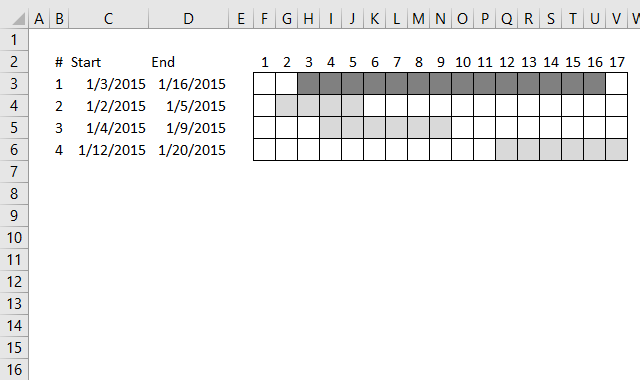For simplicity, in this example, there are only 4 date ranges in column B and C and you can see their length in the chart to the right.

Let's begin with a simple formula, it will be helpful for you if you understand this one. It calculates the number of date ranges that overlaps the first date range:

=SUMPRODUCT((B2<=C2:C5)*(C2>=B2:B5))-1

It returns 3 overlapping date ranges, which is correct. Range 2, 3 and 4 overlap range 1.

Find overlapping date ranges.

## 2. Calculate the number of overlapped ranges for all ranges in one formula?How do we calculate the number of overlapped ranges for all ranges in one formula?

=MMULT((C3:C6<=TRANSPOSE(D3:D6))*(D3:D6>=TRANSPOSE(C3:C6)),C3:C6^0)-1

This array formula returns an array: {3;2;2;1} which corresponds to the position of each date range in row 3, 4, 5, and 6.

The first date range has 3 overlapping ranges, described above. The second has 2, range 1 and 3 overlaps range 2.

The third has 2 overlapping ranges, range 1 and 2. The fourth range has 1 overlapping date range, range 1.

### Explaining formula in cell I9

#### Step 1 - Transpose array

The TRANSPOSE function converts a vertical range to a horizontal range, or vice versa.

TRANSPOSE(D3:D6)

becomes

TRANSPOSE({42020; 42009; 42013; 42024})

and returns {42020, 42009, 42013, 42024}

#### Step 2 - Logical test 1

The less than character and the equal character combined means less than or equal to.

C3:C6<=TRANSPOSE(D3:D6)

becomes

C3:C6<={42020, 42009, 42013, 42024}

becomes

{42007; 42006; 42008; 42016}<={42020, 42009, 42013, 42024}

and returns

{TRUE, TRUE, TRUE, TRUE; TRUE, TRUE, TRUE, TRUE; TRUE, TRUE, TRUE, TRUE; TRUE, FALSE, FALSE, TRUE}

#### Step 3 - Logical test 2

D3:D6>=TRANSPOSE(C3:C6)

becomes

{42020;42009;42013;42024}>={42007,42006,42008,42016}

and returns

{TRUE, TRUE, TRUE, TRUE; TRUE, TRUE, TRUE, FALSE; TRUE, TRUE, TRUE, FALSE; TRUE, TRUE, TRUE, TRUE}.

#### Step 4 - Multiply arrays

When we multiply arrays we need to make sure the size of one array matches the other array. Multiplying boolean values is the same as applying AND logic. The result is the numerical equivalent TRUE = 1 and FALSE = 0 (zero).

TRUE * TRUE = 1, TRUE * FALSE = 0 (zero) and, FALSE * FALSE = 0 (zero).

(C3:C6<=TRANSPOSE(D3:D6))*(D3:D6>=TRANSPOSE(C3:C6))

becomes

{TRUE, TRUE, TRUE, TRUE; TRUE, TRUE, TRUE, TRUE; TRUE, TRUE, TRUE, TRUE; TRUE, FALSE, FALSE, TRUE}*{TRUE, TRUE, TRUE, TRUE; TRUE, TRUE, TRUE, FALSE; TRUE, TRUE, TRUE, FALSE; TRUE, TRUE, TRUE, TRUE}

and returns {1, 1, 1, 1; 1, 1, 1, 0; 1, 1, 1, 0; 1, 0, 0, 1}.

#### Step 5 - Create an array containing 1

Exponentiation is a mathematical operation, use the ^ character or the POWER function to calculate the result of exponentiation.

When a number is raised to the power of 0 (zero) the result is always 1.

C3:C6^0

becomes

{42007;42006;42008;42016}^0

and returns {1;1;1;1}.

#### Step 6 - Evaluate MMULT function

The MMULT function calculates the matrix product of two arrays, an array as the same number of rows as array1 and columns as array2.

MMULT(array1array2)

MMULT((C3:C6<=TRANSPOSE(D3:D6))*(D3:D6>=TRANSPOSE(C3:C6)),C3:C6^0)

becomes

MMULT({1, 1, 1, 1; 1, 1, 1, 0; 1, 1, 1, 0; 1, 0, 0, 1}, {1;1;1;1})

and returns {4; 3; 3; 2}.

#### Step 7 - Subtract with 1

The MMULT function calculates all overlapping ranges even the range itself. We need to subtract with 1 to get the correct result.

MMULT((C3:C6<=TRANSPOSE(D3:D6))*(D3:D6>=TRANSPOSE(C3:C6)),C3:C6^0)-1

becomes

{4; 3; 3; 2}-1

and returns {3; 2; 2; 1}.

## 2.1 Use a drop-down list to select a date range to see overlapping date ranges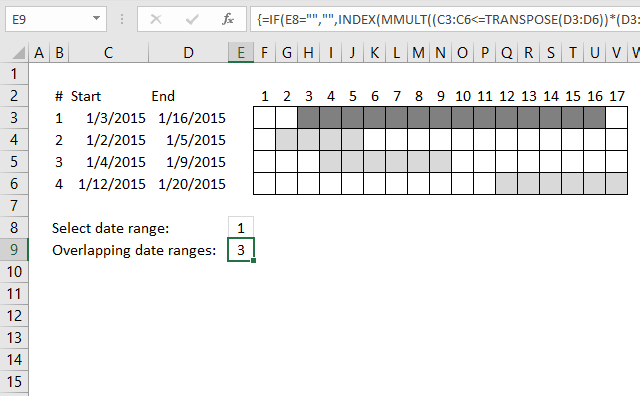In the animated picture above the drop-down list in cell D7 allows you to select a date range, cell D8 tells you how many overlapping date ranges the selected date range has.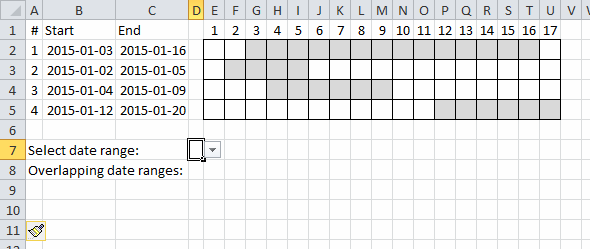I applied conditional formatting to easily spot the selected date range.

Array formula in cell D8:

=IF(D7="","",INDEX(MMULT((B2:B5<=TRANSPOSE(C2:C5))*(C2:C5>=TRANSPOSE(B2:B5)),B2:B5^0)-1,D7))

## 3. Find most overlapped date range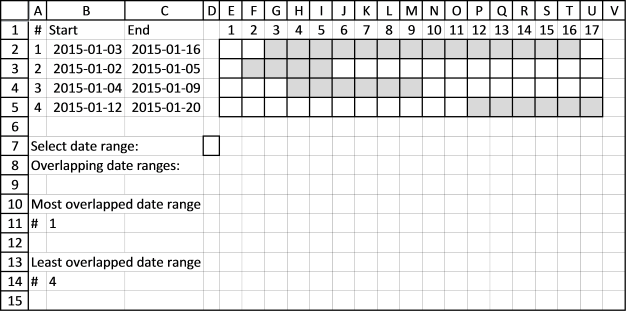Using this technique we can now construct a formula that finds the most overlapped date range, array formula in cell B11:

=INDEX(B3:B6,MATCH(MIN(MMULT((C3:C6<=TRANSPOSE(D3:D6))*(D3:D6>=TRANSPOSE(C3:C6)),C3:C6^0)-1),MMULT((C3:C6<=TRANSPOSE(D3:D6))*(D3:D6>=TRANSPOSE(C3:C6)),C3:C6^0)-1,0))

Want to know more about the excel functions used in the formulas above?
SUMPRODUCT, MMULT, INDEX, MATCH

## 4. Least overlapped date rangeArray formula in cell B14:

=INDEX(B3:B6, MATCH(MIN(MMULT((C3:C6<=TRANSPOSE(D3:D6))*(D3:D6>=TRANSPOSE(C3:C6)),C3:C6^0)-1), MMULT((C3:C6<=TRANSPOSE(D3:D6))*(D3:D6>=TRANSPOSE(C3:C6)),C3:C6^0)-1, 0))

## 5. Most overlapped date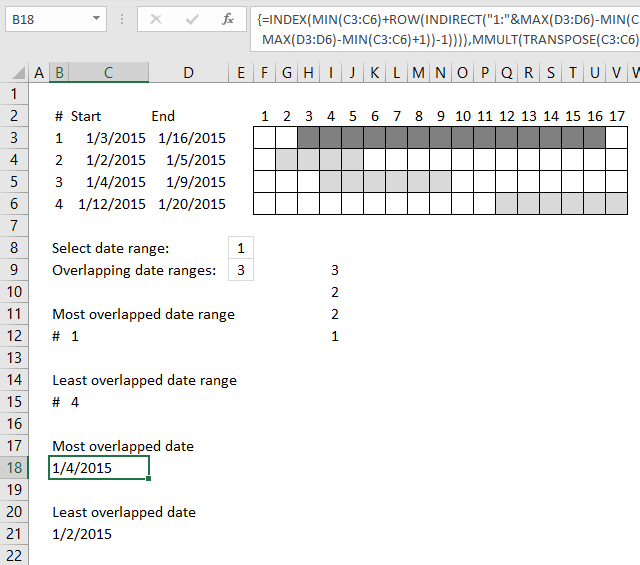Array formula in cell C18:

=INDEX(MIN(C3:C6)+ROW(INDIRECT("1:"&MAX(D3:D6)-MIN(C3:C6)+1))-1, MATCH(MAX(MMULT(TRANSPOSE(C3:C6)^0, (C3:C6<=TRANSPOSE(MIN(C3:C6)+ROW(INDIRECT("1:"&MAX(D3:D6)-MIN(C3:C6)+1))-1))*(D3:D6>=TRANSPOSE(MIN(C3:C6)+ROW(INDIRECT("1:"&MAX(D3:D6)-MIN(C3:C6)+1))-1)))), MMULT(TRANSPOSE(C3:C6)^0, (C3:C6<=TRANSPOSE(MIN(C3:C6)+ROW(INDIRECT("1:"&MAX(D3:D6)-MIN(C3:C6)+1))-1))*(D3:D6>=TRANSPOSE(MIN(C3:C6)+ROW(INDIRECT("1:"&MAX(D3:D6)-MIN(C3:C6)+1))-1))), 0))

## 6. Least overlapped date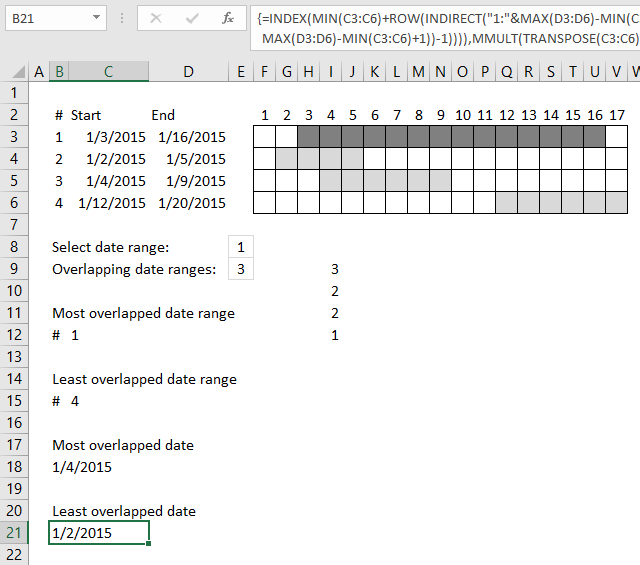Array formula in cell C21:

=INDEX(MIN(C3:C6)+ROW(INDIRECT("1:"&MAX(D3:D6)-MIN(C3:C6)+1))-1, MATCH(MIN(MMULT(TRANSPOSE(C3:C6)^0, (C3:C6<=TRANSPOSE(MIN(C3:C6)+ROW(INDIRECT("1:"&MAX(D3:D6)-MIN(C3:C6)+1))-1))*(D3:D6>=TRANSPOSE(MIN(C3:C6)+ROW(INDIRECT("1:"&MAX(D3:D6)-MIN(C3:C6)+1))-1)))), MMULT(TRANSPOSE(C3:C6)^0, (C3:C6<=TRANSPOSE(MIN(C3:C6)+ROW(INDIRECT("1:"&MAX(D3:D6)-MIN(C3:C6)+1))-1))*(D3:D6>=TRANSPOSE(MIN(C3:C6)+ROW(INDIRECT("1:"&MAX(D3:D6)-MIN(C3:C6)+1))-1))), 0))